GeeksforGeeks App
Open AppBrowser
Continue

# How to Get Length of Array in Excel VBA?

We use UBound and LBound functions to get the length of an Array in Excel VBA. In this article, we will discuss them in detail.

Syntax: UBound() function

`UBound (arrayname, [ dimension ])`

Parameters:

• arrayname: required. Array variable name
• dimension: optional

Returns: Return upper limit of an array dimension.

Syntax: LBound() Function

`LBound (arrayname, [ dimension ])`

Parameters:

• arrayname : required. Array variable name
• dimension : optional

Returns: Return lower limit of an array dimension

Sample Data: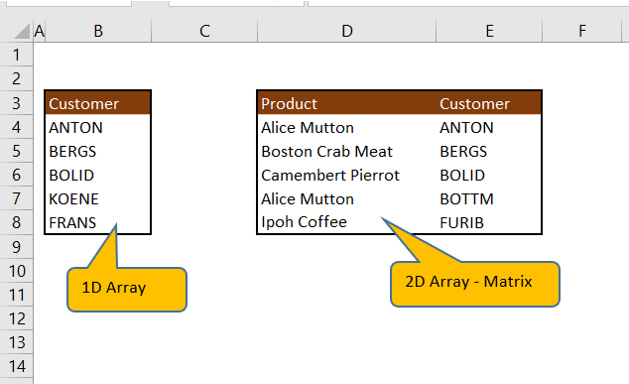### VBA Code to get the length of Array (one-dimensional array):

Declare Variables:

Declaring a customer array with the size of 10.

```Sub oneDimArrayLength()
' Array variable Declaration
Dim customer (1 To 10) As String```

Assign values to array elements

```customer(1) = "ANTON"
customer(2) = "BERGS"
customer(3) = "BOLID"
customer(4) = "KOENE"
customer(5) = "FRANS"```

Use UBound function to get the size of an array and Message box to display the result

```'Message box to popup length of 1D array
MsgBox "Array has " & UBound(customer) & " element(s)."

End Sub```

To Run VBA Code

Press Alt+F8 to popup macro window.  Select ” oneDimArrayLength” and Click Run button.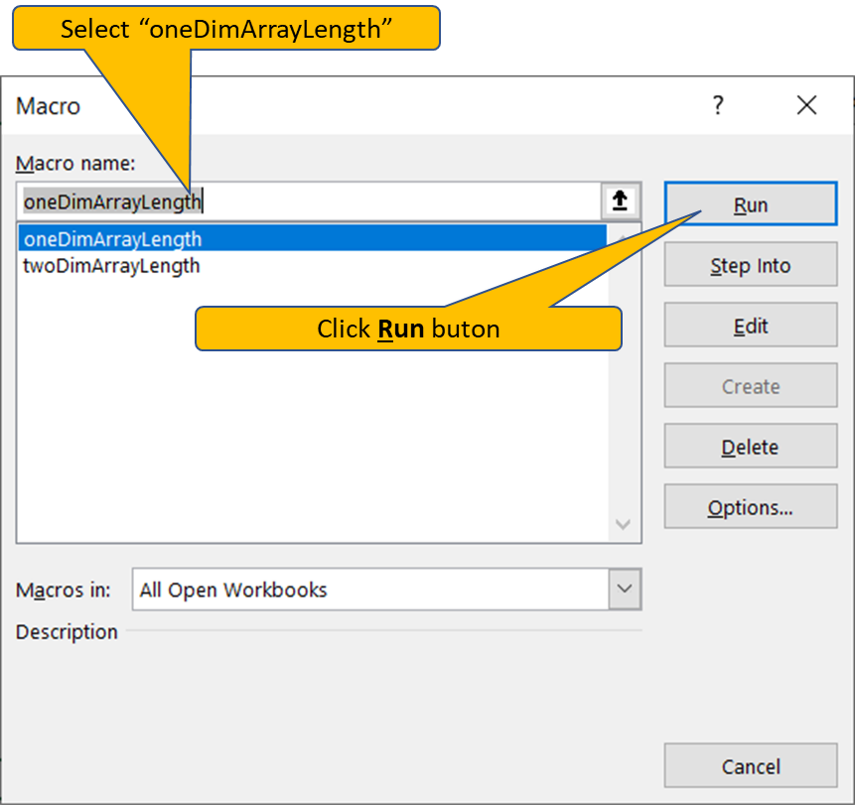Output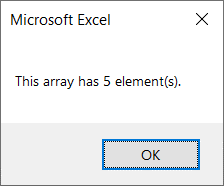VBA Code to get the length of Array (multi-dimensional array)

Declaring variables:

Declaring ProdAndCustomer multi-dimensional array size of 10 rows and 2 columns

```Sub twoDimArrayLength()

' Array variable Declaration
Dim ProdAndCustomer(1 To 10, 1 To 2) As String, noOfRow As Integer, noOfCol As Integer, noOfElements As Integer```

Assign values to array elements

```ProdAndCustomer(1, 1) = "Alice Mutton"
ProdAndCustomer(2, 1) = "Boston Crab Meat"
ProdAndCustomer(3, 1) = "Camembert Pierrot"
ProdAndCustomer(4, 1) = "Alice Mutton"
ProdAndCustomer(5, 1) = "Ipoh Coffee"

ProdAndCustomer(1, 2) = "ANTON"
ProdAndCustomer(2, 2) = "BERGS"
ProdAndCustomer(3, 2) = "BOLID"
ProdAndCustomer(4, 2) = "BOTTM"
ProdAndCustomer(5, 2) = "FURIB"```

Compute Number of Rows, Number of Columns using UBound and LBound function.  Multiply by noOfRow and noOfCol variable to get Number of elements in multi-dimensional array.

```noOfRow = UBound(ProdAndCustomer, 1) - LBound(ProdAndCustomer, 1) + 1
noOfCol = UBound(ProdAndCustomer, 2) - LBound(ProdAndCustomer, 2) + 1
noOfElements = noOfRow * noOfCol```

Message box to popup result

```'Message box to popup length of 1D array
MsgBox "Array has " & noOfElements & " element(s)."

End Sub```

To Run VBA Code

Press Alt+F8 to popup macro window.  Select ” twoDimArrayLength” and Click Run button.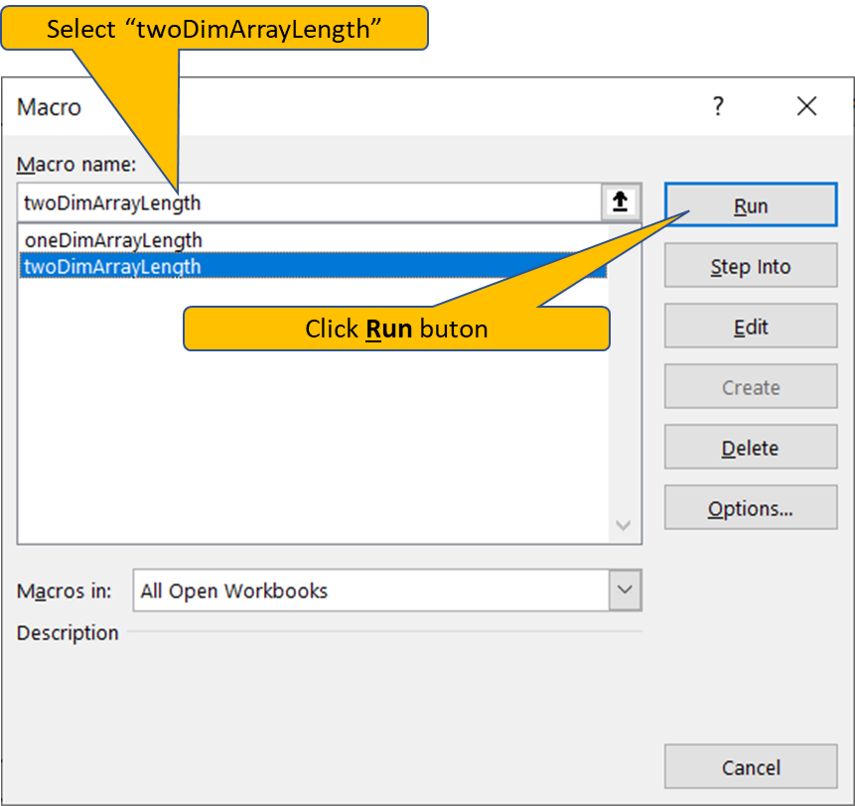Output: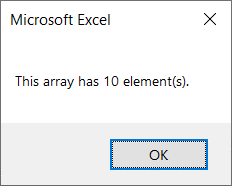My Personal Notes arrow_drop_up